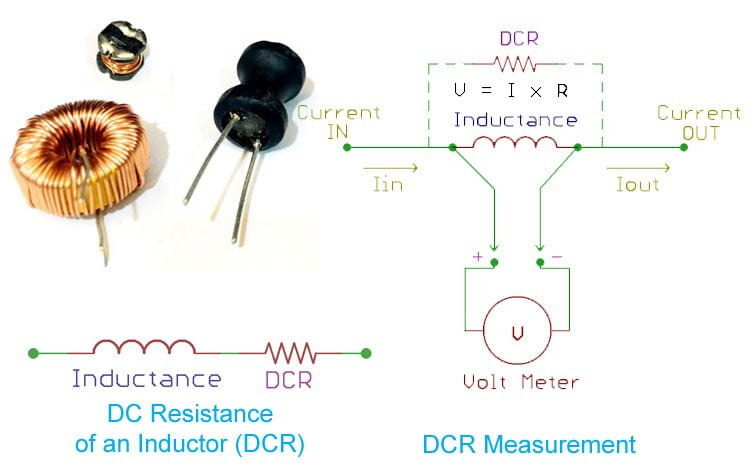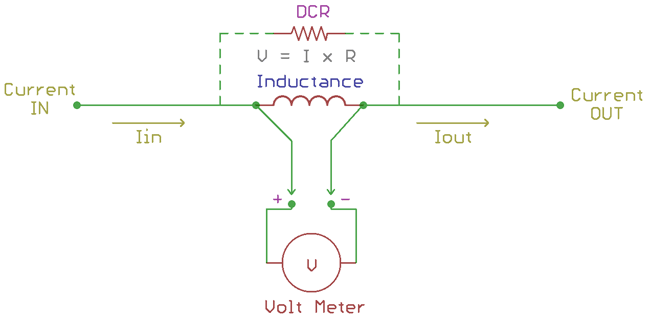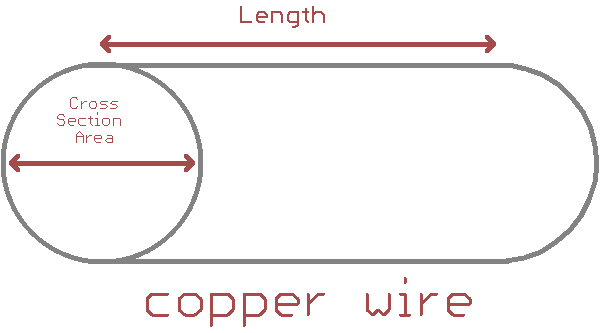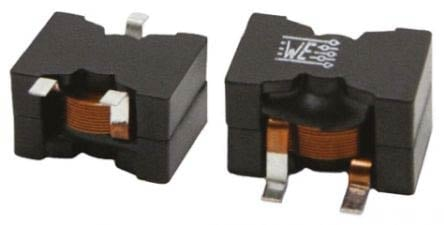# What is DCR in Inductors and how does it affect your circuit design

Published  June 26, 2019   0Inductors are widely used passive components in electronics after resistors and capacitors. An ideal inductor stores energy in a magnetic field and delivers a smooth output current to the load. But in a practical circuit, an inductor also contains some low value resistance associated with its inductance property. During the flow of DC supply or to be specific in 0 Hertz frequency, Inductors provide resistance to the flow of current. This DC resistance is referred to as DCR which stands for DC resistance. In this tutorial we will learn more about DCR and how it affects the performance of a circuit. We will also learn how to measure the DCR value of an inductor and how to reduce DCR value of an inductor during its construction.

Similar to DCR for Inductors, the capacitors also have some non-ideal parameter associated with it called Equivalent Series resistance (ESR) and Equivalent series Inductance (ESL) you can read the article on ESR and ESL in Capacitors to know more about it and their importance in circuit design.

### What is DCR in Inductors?

The term DCR stands for DC Resistance. This value represents the amount of resistance an inductor can offer when a DC signal of 0Hz is passed through it. In practice all inductors will have a small value of DCR associated with it.

The below image represents a practical Inductor with its actual inductance in series with a small DC resistance (DCR). The inductor symbol here is representing the inductance and the resistor in series with it is the DC resistance of the Inductor. In principle Inductors provide a very low resistance for DC current with low frequency and provides high resistance for high frequency inputs.The DCR of an inductor is due to the resistance of the coil using which the inductor is made. The resistance of the coil is proportional to the length of the wire used to form the coil, and the length of coil is also proportional to the inductance value of the Inductor. Hence, higher value inductors impose high resistance and low-value inductors provide low resistance. A large value of inductance requires higher winding numbers than the low-value inductors, thus increasing the copper wire length. The DCR of the Inductors typically ranges from far less than the 1 Ohms to 3-4 Ohms.

### Practical Importance of DCR

Now we know that inductors have a small value of resistance with it, but what is the problem with that? Why is it important to consider this small value of resistance while designing our circuit?

The DCR being a resistor dissipates heat and reduces efficiency just like any other resistor with a voltage drop across it. The efficiency is measured using the below formula

`Q = w (L/R)`

Where, Q is called the Q-factor. L is the inductive reactor and R is the resistance of the Inductor at a particular frequency. The ratio of an inductive reactance with the resistance at a given frequency is called the Q-factor. This Q factor is essential in various applications. Higher the Q factor is, higher will be the efficiency. If theoretically calculated, an ideal inductor has a higher Q factor compared with the real one. In real Inductors, this Q factor is dependable in DCR.

Application wise, Inductors with a high value of Q factor are used in the RF circuits where a capacitor is used in parallel with it to form a resonant tank circuit. In such a case, the high value of the Q factor of an inductor helps to balance the upper and lower frequency of the resonant circuit operating in a continuous band frequency.

In power electronics related application, the low value of DCR is essential for less power dissipation as well as small package footprints. Inductor with low DCR will have low form factor than the Inductors with the high value of DCR. The major effect of inductor’s DCR is the power dissipation due to the coil resistance. The power dissipation can be calculated by the power law P = I2R where the R is equivalent of Inductors DC resistance and I is the current flowing through it.

### How to measure DCR of an Inductor?

Most people measure the DC Resistance (DCR) of an Inductor by connecting a standard multi-meter across the Inductor leads to measure the resistance of the copper wire. It might work fair enough for large value inductors, because the copper wire there is large enough to produce a high DCR value that can be measured by the typical multi-meter resolution.

But, for a smaller value inductor the DC resistance value is too small (typically in mili-ohms range) to be measured by the standard low-cost multi-meters. Also Multi-meter’s probe wires also have DC resistance which adds up to DCR value resulting in faulty reading. So, there is a generic problem in Inductor’s DCR measurement.

The actual way to measure an Inductor’s DCR value is by using a Kelvin sensing path across the leads and applying current across the Inductor. As the DCR of Inductor is the DC resistance of the copper wire, it will produce a voltage across the Inductor’s terminal based on the Ohms law, V = I x R. This voltage can be measured using the multi-meter. Obviously, this measurement technique has a limitation. Before taking the measurement one should need to be aware about a few things which are listed below.

1. The maximum current rating of the Inductors. Current should not be exceeding the maximum current rating stated in the Inductor’s datasheet.
2. A breadboard is not suitable for Inductors DCR measurement as the breadboard connection also contributes to noise and resistance.
3. It is good to use proper PCB with only test points, Current in and out connectors and the component pads component holding fixture to avoid soldering.

The below image shows the circuit to measure DCR value of an Inductor. The Inductor shown here is an ideal Inductor and the DC Resistance is the equivalent series resistance. The sense line is the Kelvin sense lines.Let’s assume that the Inductor used here has a continuous current rating of 1A. So we the input current here will be 1A. Higher the value of input current higher will be the resolution of the measured DCR value, but if your inductor cannot handle high current low value currents can also be used.

After passing the current the voltage drop across the leads of the inductor has to be measured. Suppose the voltage drop across the inductor is calculated at approx 50mV. Then, the DCR of that inductor can be calculated as

```V = I x R
R = V / I
R = 0.05 / 1
R = 0.05 ohm```

### How to reduce the DCR while constructing the Inductor

The DCR value of an inductor has no significant advantage and hence it is always better to select an Inductor with low DCR value. Normally when Inductors are being constructed or designed, the DCR parameter is also considered. DCR of an inductor needs to be very low so that the inductor does not block the DC current flow. The following techniques are used to reduce the DCR value of a inductor

1. The resistance depends on the copper wire length and thickness. To lower the DC resistance of an inductor, instead of a single wire, multiple wires can be wounded in parallel. Due to this connection, the resulting resistance becomes less. Consider a single copper wire with some x value of resistance. If multiple such wires are connected in parallel, the equivalent resistance will be reduced since resistors in parallel will have a low equivalent resistance as output.

2. Increasing the cross-sectional area of the copper wire decreases the DC resistance of the inductors. Therefore, thicker wires are beneficial for reduced DCR.3. Another technique is to use flat copper wire instead of the round copper wires. Flat wires have a large area compared with the round wires. This is also beneficial to reduce the overall resistance.
The below image is an inductor which is constructed using flat wire. The manufacturer is Wurth Electronics and the part number is 7443641000. As per the datasheet, the inductor has an inductance of 10uH and the DC resistance is 2.4 mili-ohms at 20 degree Celsius.4. Inductor datasheet provides the ratings of the inductor where maximum DCR value is specified. This value varies based on temperature. It is advised to use the inductor in given ambient temperature condition to operate them in the minimum DCR value region.

So DCR of  an inductor is an important factor and should be considered while designing any circuit.